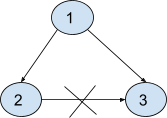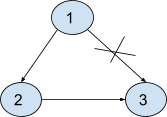3

# Redundant Connection - II

Difficulty: MEDIUMContributed By
Deep Mavani
Avg. time to solve
20 min
Success Rate
80%

Problem Statement

#### Note:

``````1. A rooted tree is a directed graph such that, there is exactly one node (the root) for which all other nodes are descendants of this node, plus every node has exactly one parent, except for the root node which has no parents.
2. If there are multiple answers, return the answer that occurs last in the given 2D-array. The answer edge [u, v] should be in the same format, with u < v.
``````
##### Example:
``````Let’s say we have 3 edges that are {1 , 2} , {1 , 3} and {2 , 3}. So the resulting graph from these edges will be :
````````````If we remove the edge {2, 3} then the resulting graph will be a tree
with 'N' nodes.
``````
##### Input format:
``````The very first line of input contains an integer ‘T’ denoting the number of test cases.

The first line of every test case contains an integer ‘N’ denoting the number of nodes of the graph.

The second line of every test case contains a 2D array of 'N' X 2 size which contains 'N' edges of the graph.
``````
##### Output format:
``````For each test case, return that single edge that if removed, the resulting graph will be a tree of 'N' nodes.

Output for each test case is printed on a separate line.
``````
##### Note:
``````1. You do not need to print anything, it has already been taken care of. Just return an edge that can be removed so that the resulting graph is a tree of N nodes.
``````
##### Constraints:
``````1 <= T <= 10
3 <= N <= 1000
1 <= 'ARR[i]' <= N

Where ‘ARR[i]’ represents the edges of the graph.

Time Limit: 1 sec
``````
##### Sample Input 1:
``````2
3
1 2
1 3
2 3
3
1 2
2 3
1 3
``````
##### Sample Output 1:
``````2 3
1 3
``````
##### Explanation for sample input 1:
``````In test case 1, It is already explained above in the example.

In test case 2, Let’s say we have 3 edges that are {1 , 2} , {2 , 3} and {1 , 3}. So the resulting graph from these edges will be :
````````````If we remove the edge {1, 3} then the resulting graph will be a tree with 'N' nodes.
``````
##### Sample Input 2:
``````2
5
1 2
2 3
3 4
4 1
1 5
4
1 2
1 3
3 4
1 4
``````
##### Sample Output 2:
`````` 4 1
1 4
``````Console